# Probabilities in Keno

To understand keno probabilities you must first fully understand the combinatorial function. For example, in the Maryland lotto the player picks 6 numbers out of 49. Then the lottery will draw 6 numbers out of 49, without replacement. The player wins the jackpot if all six numbers match (order does not matter). If you don’t know the probability of winning per game is 1 in 13,983,816 then you need to review the combinatorial function before going further.

In keno the casino, or game machine, will draw 20 numbers out of 80, without replacement. Before this happens the player may pick 1 to 15 (or more) numbers. A "catch" is a number the player picked and was drawn by the casino. A "miss" is a number of the player picked but was not drawn by the casino. The player is paid according to the number of picks made and catches.

Although the player picks first the combinations get very huge when calculation the number of ways the casino can match the player’s picks. To start with there are combin(80,20) = 3,535,316,142,212,180,000 ways the casino can draw 20 numbers out of 80. Many calculators don’t support numbers this big. So to keep the combinations smaller we will assume the casino draws first, but keeps the numbers secret, and player tries to match the numbers already drawn. I assure you the math is the same either way.

All the following images were taken from the Luxor keno rulebook. All pays are on a "for one" basis. In other words the player never gets his original bet back, even if he wins.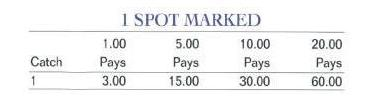## 1 Spot

Let’s examine the 1 Spot card first. Here the player just picks one number, from 1 to 80. Then the casino will draw 20 numbers. If one of these 20 match the player’s number then the player is paid \$3 for a \$1 bet.

There are 80 numbers the player can pick. 20 of these will result in a winning ticket. So the probability of winning is 20/80 = ¼. The expected return is thus ¼ * 3 = 75%.

## 2 Spot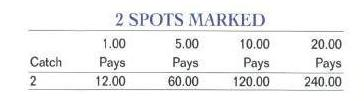We need to start using the combinatorial function for the 2 Spot card. The player picks 2 numbers and casino must draw both of them for the player to win. There is no consolation prize for only catching one. There are a total of combin(80,2) = 3160 ways the player can draw 2 numbers out of 80. However the player must draw 2 out of the casino’s 20 drawn numbers to win. So there are only combin(20,2) = 190 ways to draw 2 winning numbers. So the probability of winning is 190/3160 = 6.01%. A winning \$1 ticket pays \$12, so the expected return is (190/3160) * \$12 = 72.15%.

Alternatively, the probability the player’s first pick will match one of the casino’s numbers is 20/80. If it does match the probability the second pick will match one of the casino’s other 19 numbers out of 79 is 19/79. So the probability of winning is (20/80)*(19/79) = 6.01%.

## 3 Spot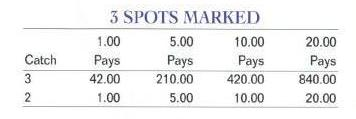With the 3 Spot card we start having consolation prizes for not matching every pick. First let’s determine the probability of catching all 3 marks. There are combin(80,3) = 82,160 ways to draw 3 numbers out of 80. To catch all three the player must pick all 3 numbers from the casino’s 20 drawn numbers. There are combin(20,3) = 1140 ways to do this. So the probability of catching all 3 is 1140/82160 = 1.39%.

There is a consolation prize for catching 2 out of 3. Imagine the casino puts the 20 drawn numbers in a winning urn and the other 60 numbers in a losing urn. The number of ways to catch 2 out of 3 is the number of ways to draw 2 balls from the 20 in the winning urn, which is combin(20,2) = 190. The number of ways to draw one losing ball out of 60 is obviously 60. So the number of ways to catch 2 out of 3 is the product of the number of ways to pick 2 winning balls from the winning urn and 1 ball from the losing urn, or 190*60 = 11,400. We already know there are combin(80,3) = 82,160 total ways to draw 3 balls out of 80. So the probability of catching 2 out of 3 is 11,400/82,160 = 13.88%.

For any bet the expected return is the dot product over every possible event of the probability and what it pays. The following table shows every possible outcome, with the total in the bottom row.

 Catch Formula Combinations Pays Return 3 combin(20,3)*combin(60,0) 1140 \$42 \$47880 2 combin(20,2)*combin(60,1) 11400 \$1 \$11400 1 combin(20,1)*combin(60,2) 35400 \$0 \$0 0 combin(20,0)*combin(60,3) 34220 \$0 \$0 Total 82160 \$ \$59280

The bottom right cell shows that over all 82,160 combinations the player will get back \$59,280. So the expected return is 59280/82160 = 72.15%.

## 4 Spot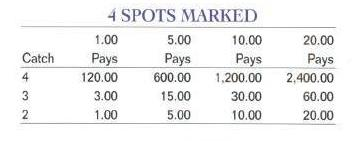For the 4 Spot ticket the number of ways to catch all four is combin(20,4) = 4845. The number of ways to catch 3 and miss one is the product of the number of ways to choose 3 out of 20 and the number of ways to choose 1 out of 60, or combin(20,3) * 60 = 1140*60 = 68,400. The number of ways to catch 2 and miss 2 is the product of the number of watch to choose 2 out of 20 winners and 2 out of 60 losers, or combin(20,2)*combin(60,2) = 190 * 1770 = 336,300. The following table shows all the possible outcomes.

 Catch Formula Combinations Pays Return 4 combin(20,4)*combin(60,0) 4845 \$120 \$581400 3 combin(20,3)*combin(60,1) 68400 \$3 \$205200 2 combin(20,2)*combin(60,2) 336300 \$1 \$336300 1 combin(20,1)*combin(60,3) 684400 \$0 \$0 0 combin(20,0)*combin(60,4) 487635 \$0 \$0 Total 1581580 \$ \$1122900

The return of the 4 Spot ticket is 1,122,900/1,581,580 = 71.00%.

## 4 Spot Special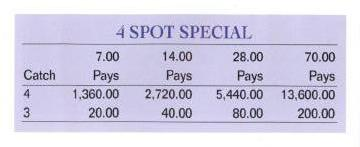Next, let’s look at the 4 Spot Special. We just worked out all the combinations, so we only need to apply them to a different pay table as follows, based on a \$7 ticket.

 Catch Formula Combinations Pays Return 4 combin(20,4)*combin(60,0) 4845 \$1360 \$6589200 3 combin(20,3)*combin(60,1) 68400 \$20 \$1368000 2 combin(20,2)*combin(60,2) 336300 \$0 \$0 1 combin(20,1)*combin(60,3) 684400 \$0 \$0 0 combin(20,0)*combin(60,4) 487635 \$0 \$0 Total 1581580 \$ \$7957200

The expected return of the ticket is 7,957,200/1,581,580 = \$5.0312. However we must divide this by \$7, the cost of the ticket, to get the expected return, which is \$5.0312/\$7 = 71.87%. So, if you don’t mind extra volatility, and were going to bet \$7 anyway, the 4 Spot Special is a better bet than the regular 4 Spot.

## 5 Spot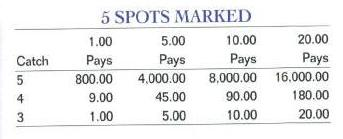For the rest of the tickets I will present just the return tables. Following is the return table for the 5 Spot.

 Catch Formula Combinations Pays Return 5 combin(20,5)*combin(60,0) 15504 \$800 \$12403200 4 combin(20,4)*combin(60,1) 290700 \$9 \$2616300 3 combin(20,3)*combin(60,2) 2017800 \$1 \$2017800 2 combin(20,2)*combin(60,3) 6501800 \$0 \$0 1 combin(20,1)*combin(60,4) 9752700 \$0 \$0 0 combin(20,0)*combin(60,5) 5461512 \$0 \$0 Total 24040016 \$ \$17037300

5-Spot return = \$17,037,300/24,040,016 = 70.87%

## 6 Spot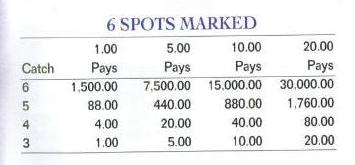Catch Formula Combinations Pays Return 6 combin(20,6)*combin(60,0) 38760 \$1500 \$58140000 5 combin(20,5)*combin(60,1) 930240 \$88 \$81861120 4 combin(20,4)*combin(60,2) 8575650 \$4 \$34302600 3 combin(20,3)*combin(60,3) 39010800 \$1 \$39010800 2 combin(20,2)*combin(60,4) 92650650 \$0 \$0 1 combin(20,1)*combin(60,5) 109230240 \$0 \$0 0 combin(20,0)*combin(60,6) 50063860 \$0 \$0 Total 300500200 \$ \$213314520

6-Spot return = 70.99%

## 7 Spot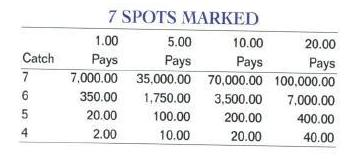Catch Formula Combinations Pays Return 7 combin(20,7)*combin(60,0) 77520 \$7000 \$542640000 6 combin(20,6)*combin(60,1) 2325600 \$350 \$813960000 5 combin(20,5)*combin(60,2) 27442080 \$20 \$548841600 4 combin(20,4)*combin(60,3) 165795900 \$2 \$331591800 3 combin(20,3)*combin(60,4) 555903900 \$0 \$0 2 combin(20,2)*combin(60,5) 1037687280 \$0 \$0 1 combin(20,1)*combin(60,6) 1001277200 \$0 \$0 0 combin(20,0)*combin(60,7) 386206920 \$0 \$0 Total 3176716400 \$ \$2237033400

The 7-spot has a return of \$2237033400/3176716400 = 70.42%

## 8 Spot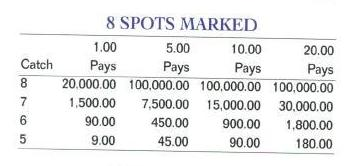Catch Formula Combinations Pays Return 8 combin(20,8)*combin(60,0) 125970 \$20000 \$2519400000 7 combin(20,7)*combin(60,1) 4651200 \$1500 \$6976800000 6 combin(20,6)*combin(60,2) 68605200 \$90 \$6174468000 5 combin(20,5)*combin(60,3) 530546880 \$9 \$4774921920 4 combin(20,4)*combin(60,4) 2362591575 \$0 \$0 3 combin(20,3)*combin(60,5) 6226123680 \$0 \$0 2 combin(20,2)*combin(60,6) 9512133400 \$0 \$0 1 combin(20,1)*combin(60,7) 7724138400 \$0 \$0 0 combin(20,0)*combin(60,8) 2558620845 \$0 \$0 Total 28987537150 \$ \$20445589920

8-Spot return = \$20445589920/28987537150 = 70.53%.

## 9 Spot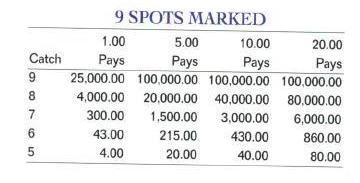Catch Formula Combinations Pays Return 9 combin(20,9)*combin(60,0) 167960 \$25000 \$4199000000 8 combin(20,8)*combin(60,1) 7558200 \$4000 \$30232800000 7 combin(20,7)*combin(60,2) 137210400 \$300 \$41163120000 6 combin(20,6)*combin(60,3) 1326367200 \$43 \$57033789600 5 combin(20,5)*combin(60,4) 7560293040 \$4 \$30241172160 4 combin(20,4)*combin(60,5) 26461025640 \$0 \$0 3 combin(20,3)*combin(60,6) 57072800400 \$0 \$0 2 combin(20,2)*combin(60,7) 73379314800 \$0 \$0 1 combin(20,1)*combin(60,8) 51172416900 \$0 \$0 0 combin(20,0)*combin(60,9) 14783142660 \$0 \$0 Total 231900297200 \$ \$162869881760

9 Spot Return = 70.23%

## 10 Spot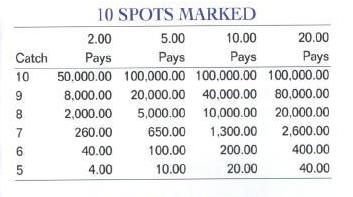For some reason there is a \$2 minimum beginning with the 10-spot. Here is the return table for a \$2 ticket.

 Catch Formula Combinations Pays Return 10 combin(20,10)*combin(60,0) 184756 \$50000 \$9237800000 9 combin(20,9)*combin(60,1) 10077600 \$8000 \$80620800000 8 combin(20,8)*combin(60,2) 222966900 \$2000 \$445933800000 7 combin(20,7)*combin(60,3) 2652734400 \$260 \$689710944000 6 combin(20,6)*combin(60,4) 18900732600 \$40 \$756029304000 5 combin(20,5)*combin(60,5) 84675282048 \$4 \$338701128192 4 combin(20,4)*combin(60,6) 242559401700 \$0 \$0 3 combin(20,3)*combin(60,7) 440275888800 \$0 \$0 2 combin(20,2)*combin(60,8) 486137960550 \$0 \$0 1 combin(20,1)*combin(60,9) 295662853200 \$0 \$0 0 combin(20,0)*combin(60,10) 75394027566 \$0 \$0 Total 1646492110120 \$ \$2320233776192

The \$2 10-spot returns on average \$1.4092. So the expected return is \$1.4092/2 = 70.46%.

The Luxor keno rule book goes through a pick 15, plus a pick 20. However hopefully you understand how to do the math yourself by now.